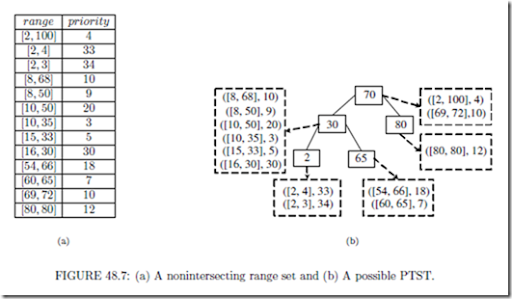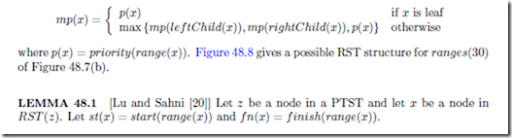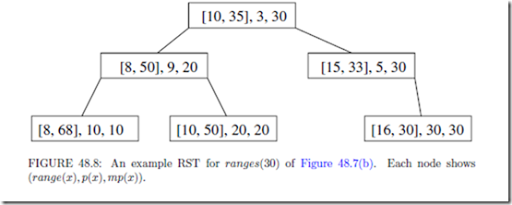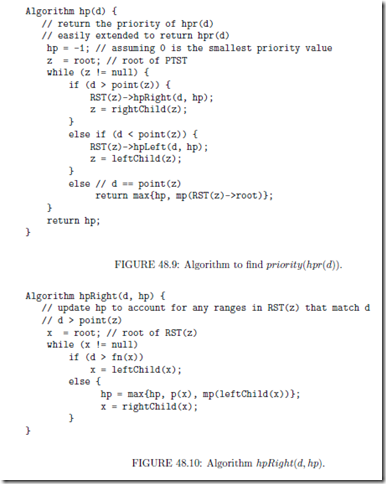# IP Router Tables:Highest-Priority Matching

##### Highest-Priority Matching

The trie data structure may be used to represent a dynamic preﬁx-router-table in which the highest-priority tie-breaker is in use. Using such a structure, each of the dynamic router- table operations may be performed in O(W ) time. Lu and Sahni  have developed the binary tree on binary tree (BOB) data structure for highest-priority dynamic router-tables.

Using BOB, a lookup takes O(log2 n) time and cache misses; a new rule may be inserted and an old one deleted in O(log n) time and cache misses. Although BOB handles ﬁlters that are non-intersecting ranges, specialized versions of BOB have been proposed for preﬁx ﬁlters.

Using the data structure PBOB (preﬁx BOB), a lookup, rule insertion and deletion each take O(W ) time and cache misses. The data structure LMPBOB (longest matching-preﬁx BOB) is proposed in  for dynamic preﬁx-router-tables that use the longest matching- preﬁx rule. Using LMPBOB, the longest matching-preﬁx may be found in O(W ) time and O(log n) cache misses; rule insertion and deletion each take O(log n) time and cache misses. On practical rule tables, BOB and PBOB perform each of the three dynamic-table operations in O(log n) time and with O(log n) cache misses. Other data structures for maximum-priority matching are developed in [12, 13].

The Data Structure BOB

The data structure binary tree on binary tree (BOB) comprises a single balanced binary search tree at the top level. This top-level balanced binary search tree is called the point search tree (PTST). For an n-rule NHRT, the PTST has at most 2n nodes (we call this the PTST size constraint). With each node z of the PTST, we associate a point, point(z). The PTST is a standard red-black binary search tree (actually, any binary search tree structure that supports eﬃcient search, insert, and delete may be used) on the point(z) values of its node set . That is, for every node z of the PTST, nodes in the left subtree of z have smaller point values than point(z), and nodes in the right subtree of z have larger point values than point(z).

Let R be the set of nonintersecting ranges. Each range of R is stored in exactly one of the nodes of the PTST. More speciﬁcally, the root of the PTST stores all ranges r R such that start(r) point(root) f inish(r); all ranges r R such that f inish(r) < point(root) are stored in the left subtree of the root; all ranges r R such that point(root) < start(r)

(i.e., the remaining ranges of R) are stored in the right subtree of the root. The ranges allocated to the left and right subtrees of the root are allocated to nodes in these subtrees using the just stated range allocation rule recursively.

For the range allocation rule to successfully allocate all r R to exactly one node of the PTST, the PTST must have at least one node z for which start(r) point(z) f inish(r).

Figure 48.7 gives an example set of nonintersecting ranges and a possible PTST for this setof ranges (we say possible, because we haven’t speciﬁed how to select the point(z) values and even with speciﬁed point(z) values, the corresponding red-black tree isn’t unique). The number inside each node is point(z), and outside each node, we give ranges(z).

Let ranges(z) be the subset of ranges of R allocated to node z of the PTST. Since the PTST may have as many as 2n nodes and since each range of R is in exactly one of the sets ranges(z), some of the ranges(z) sets may be empty.

The ranges in ranges(z) may be ordered using the < relation for non-intersecting ranges.

Using this < relation, we put the ranges of ranges(z) into a red-black tree (any balanced binary search tree structure that supports eﬃcient search, insert, delete, join, and split may be used) called the range search-tree or RST (z). Each node x of RST (z) stores exactly one range of ranges(z). We refer to this range as range(x). Every node y in the left (right) subtree of node x of RST (z) has range(y) < range(x) (range(y) > range(x)). In addition, each node x stores the quantity mp(x), which is the maximum of the priorities of the ranges associated with the nodes in the subtree rooted at x. mp(x) may be deﬁned recursively as below.1. For every node y in the right subtree of x, st(y) st(x) and f n(y) f n(x).

2. For every node y in the left subtree of x, st(y) st(x) and f n(y) f n(x).

Both BOB and BOT (the binary tree on trie structure of Gupta and McKeown ) use a range allocation rule identical to that used in an interval tree  (See Chapter 18). While BOT may be used with any set of ranges, BOB applies only to a set of non-intersecting ranges. However, BOB reduces the search complexity of BOT from O(W log n) to O(log2 n) and reduces the update complexity from O(W ) to O(log n).

Search for the Highest-Priority Matching Range

The highest-priority range that matches the destination address d may be found by following a path from the root of the PTST toward a leaf of the PTST. Figure 48.9 gives the algorithm. For simplicity, this algorithm ﬁnds hp = priority(hpr(d)) rather than hpr(d). The algorithm is easily modiﬁed to return hpr(d) instead.

We begin by initializing hp = 1 and z is set to the root of the PTST. This initialization assumes that all priorities are 0. The variable z is used to follow a path from the root toward a leaf. When d> point(z), d may be matched only by ranges in RST (z) and those in the right subtree of z. The method RST(z)->hpRight(d,hp) (Figure 48.10) updates hp to reﬂect any matching ranges in RST (z). This method makes use of the fact that d > point(z). Consider a node x of RST (z). If d > f n(x), then d is to the right (i.e., d> f inish(range(x))) of range(x) and also to the right of all ranges in the right subtree of x. Hence, we may proceed to examine the ranges in the left subtree of x. When d f n(x), range(x) as well as all ranges in the left subtree of x match d. Additional matching ranges may be present in the right subtree of x. hpLef t(d, hp) is the analogous method for the case when d < point(z).

Complexity The complexity of the invocation RST(z)->hpRight(d,hp) is readily seen to be O(height(RST (z)) = O(log n). Consequently, the complexity of hp(d) is O(log2 n). To determine hpr(d) we need only add code to the methods hp(d), hpRight(d, hp), and hpLef t(d, hp) so as to keep track of the range whose priority is the current value of hp. So, hpr(d) may be found in O(log2 n) time also.

The details of the insert and delete operation as well of the BOB variants PBOB and LMPBOB may be found in .